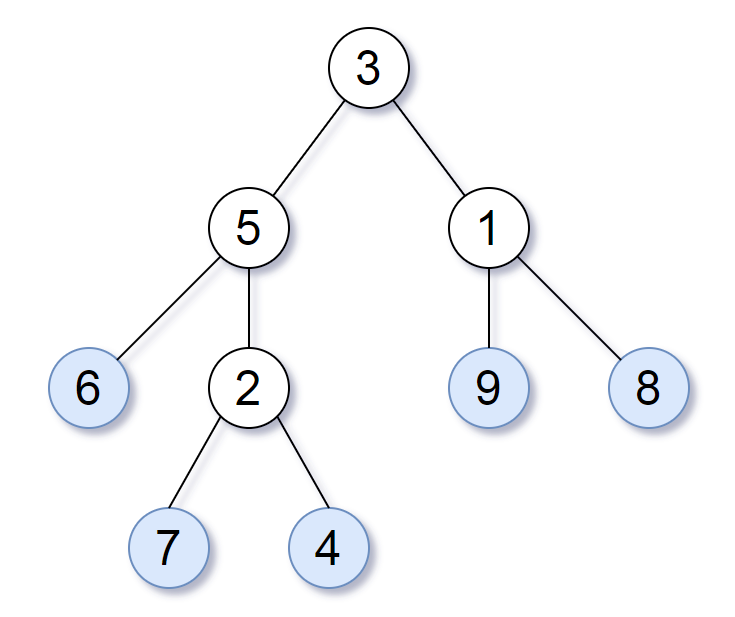# 872.leaf## Description

Consider all the leaves of a binary tree. From left to right order, the values of those leaves form a leaf value sequence.For example, in the given tree above, the leaf value sequence is (6, 7, 4, 9, 8).

Two binary trees are considered leaf-similar if their leaf value sequence is the same.

Return true if and only if the two given trees with head nodes root1 and root2 are leaf-similar.

### Note:

• Both of the given trees will have between 1 and 100 nodes.

1. updating

## 参考代码

``````Definition for a binary tree node.
class TreeNode:
def __init__(self, x):
self.val = x
self.left = None
self.right = None

class Solution:
def leafSimilar(self, root1, root2):
li1=[]
li2=[]
def _int(root):
if(root==None):
return
else:
if(root.left == None):
if(root.right == None):
li1.append(root.val)
return
else:
_int(root.right)
else:
_int(root.left)
if(root.right != None):
_int(root.right)
def _int2(root):
if(root==None):
return
else:
if(root.left == None):
if(root.right == None):
li2.append(root.val)
return
else:
_int2(root.right)
else:
_int2(root.left)
if(root.right != None):
_int2(root.right)
_int(root1)
_int2(root2)
print('li1',li1)
print('li2',li2)
if(li1 == li2):
return True
return False
``````